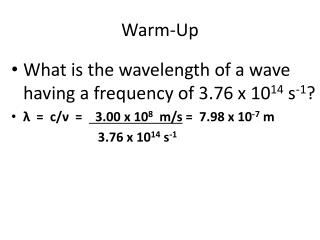# Warm-Up - PowerPoint PPT PresentationDownload PresentationWarm-Up

Warm-UpDownload Presentation## Warm-Up

- - - - - - - - - - - - - - - - - - - - - - - - - - - E N D - - - - - - - - - - - - - - - - - - - - - - - - - - -
##### Presentation Transcript

1. Warm-Up • What is the wavelength of a wave having a frequency of 3.76 x 1014 s-1? • λ = c/ν = 3.00 x 108 m/s= 7.98 x 10-7 m 3.76 x 1014 s-1

2. What is the frequency of a 6.9 x 10-13 m wave? • ν = c/λ = 3.00 x 108 m/s = 4.3 x 1020 s-1 6.9 x 10-13 m

3. What is the wavelength of a 2.99 Hz wave? • λ = c/ν = 3.00 x 108 m/s= 1.00 x 108 m 2.99 Hz

4. What is the wavelength of a 1.28 x 1017 Hz wave? • λ = c/ν = 3.00 x 108m/s 1.28 x 1017 Hz   = 2.34 x 10-9 m

5. What is the wavelength of 109.6 MHz wave? • λ = c/ν = 3.00 x 108m/s 109.6 MHz =109.6 x 106 Hz = 2.74 m

6. What is the energy of a 7.66 x 1014 Hz wave? • E = hν = 6.626 x 10-34Jsx 7.66 x 1014Hz • = 5.08 x 10-19 J

7. What is the frequency of a wave carrying 8.35 x 10-18 J of energy? • ν = E / h = 8.35 x 10-18J= 1.26 x 1016 s-1 6.626 x 10-34Js

8. What is the frequency of a 1.78 x 10-15 J wave? • ν = E / h = 1.78 x 10-15 J = 2.69 x 1018 s-1 6.626 x 10-34J-s

9. What is the energy of a 3.12 x 1018 s-1 wave? • E = h ν = 6.626 x 10-34 J-s x 3.12 x 1018s-1 = 2.07 x 10-15 J

10. What is the frequency of a 1.31 x 10-22 J wave? What is its wavelength? ν = E / h = 1.31 x 10-22 J = = 1.98 x 10+11 s-1 6.626 x 10-34J-s λ = c/ν = 3.00 x 108 m/s 1.98 x 1011 s-1 = 0.001 52 m = 1.52 x 10-3m

11. What is the wavelength of a 7.65 x 10-17 J wave? ν = E / h = 7.65 x 10-17 J = 1.15 x 1017 s-1 6.626 x 10-34J-s λ = c/ν = 3.00 x 108 m/s = 1.15 x 1017 s-1 2.61 x 10-9 m

12. What is the energy of a 9,330 cm wave? ν = c/λ = 3.00 x 108 m/sx 100 cm 9,330 cm 1 m = 3.22 x 106 s-1 E = h ν = 6.626 x 10-34 J-s x 3.22 x 106 s-1 = 2.13 x 10-27 J

13. What is the wavelength of a 1.528 x 10-13 J wave? ν = E / h = 1.528 x 10-13 J = 2.306 x 1020 s-1 6.626 x 10-34J-s λ = c/ν = 3.00 x 108m/s 2.306 x 1020 s-1 = 1.30 x 10-12 m

14. Which of the following types of radiation is not a part of the electromagnetic spectrum? • Microwave • X-ray • Beta ray • infrared

15. Which has the greatest energy? • Ultraviolet • Infrared • Green light • Blue light

16. Radiation with a longer wavelength have • A smaller frequency and higher energy • A smaller frequency and lower energy • A higher frequency and higher energy • A higher frequency and lower energy

17. Arrange the following electromagnetic radiations in order of decreasing wavelength (largest to smallest). • Infrared radiation from a heat lamp • Ultraviolet light form the sun • Dental X-rays • The signal from a short-wave radio station • Green light. 2 4 5 1 3

18. Compare the broadcast frequencies of the following two radio stations: KFGE = 98.1 MHz KBBK = 107.3 MHz Which has thefastest speed? KFGE KBBK SAME CAN’T TELL A photon of which would have the higher energy? KFGE KBBK SAME CAN’T TELL